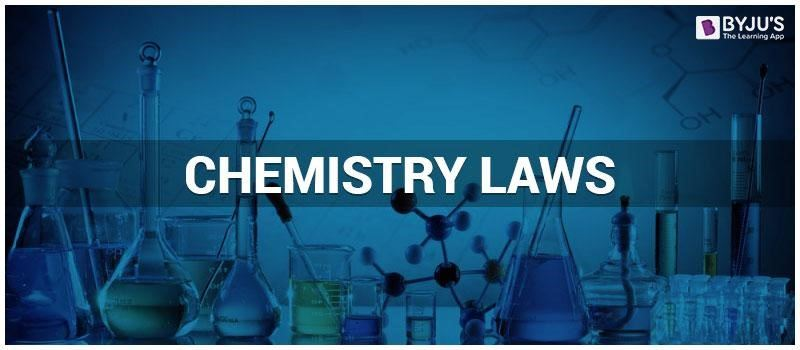# Chemistry lawsChemistry is all around us, from the air we breathe to the food we eat, chemistry is involved in it all. But then how do we make sense of all the things that are happening around us? Over the years chemists have been observing nature and the matter around us coming up with laws of chemistry which laid the foundation for us to understand further about the chemistry of our universe. Let us have a brief look at some many chemistry laws.

### Law of conservation of mass

The law of conservation of mass is often referred to as the most fundamental concept of chemistry. The law of conservation of mass states that :

 In the process of a chemical reaction, the amount of matter involved remains the same before and after the reaction occurs.

### Law of conservation of energy

The law of conservation of energy is the first basic law which concerns a thermodynamic system. The law states that:

 The total energy of a system is conserved given it is isolated from its surroundings.

### Boyle’s Law

Also known by the names of Boyle–Mariotte law or Mariotte’s law, Boyle’s law states that:

 The absolute pressure exerted by a given mass of an ideal gas is inversely proportional to the volume it occupies if the temperature and amount of gas remain unchanged within a closed system.

### Charles law

Also known as the law of volumes, Charles’s law is an experimental gas law that describes how gases tend to expand when heated. The law states that:

 When the pressure on a sample of a dry gas is held constant, the Kelvin temperature and the volume will be directly related

### Dalton’s law of partial pressure

Dalton’s law of partial pressures states that:

 In a mixture of non-reacting gases, the total pressure exerted is equal to the sum of the partial pressures of the individual gases.

This particular law was discovered in 1811 by Amedeo Avogadro. Avogadro’s Law states that :

 Equal volumes of all gases, at the same temperature and pressure, have the same number of molecules.

### Ideal Gas Law

The volume (V) occupied by n moles of any gas has a pressure (P) at a temperature (T) in Kelvin. The relationship between these variables is signifying the law is given below.

 PV = nRT

### Periodic Law

The periodic law was developed independently by Dmitri Mendeleev and Lothar Meyer in 1869. The Periodic Law states that:

 The physical and chemical properties of the elements recur in a systematic and predictable way when the elements are arranged in order of increasing atomic number.

It means, when the elements are arranged in the order of their increasing atomic numbers, it is observed that the elements of similar properties recur at regular intervals or periodically. As a result of this, the elements fall in certain groups and lead to an arrangement called the modern periodic table of elements.

### Laws of thermodynamics

The four laws of thermodynamics define the fundamental physical quantities that characterize thermodynamic systems at thermal equilibrium. The laws describe how these quantities behave under various circumstances and forbid certain phenomena. There are four laws of thermodynamics.

1. Zeroth law of thermodynamics
2. First law of thermodynamics
3. Second law of thermodynamics
4. Third law of thermodynamics

Any change in the magnetic environment of a coil of wire will cause a voltage (emf) to be “induced” in the coil. No matter how the change is produced, the voltage will be generated. This is made clear by Faraday’s Law. Faraday’s Law states that:

 The amount of substance liberated at an electrode is directly proportional to the quantity of electricity passed.

### Henry’s Law

Henry’s law is one of the gas laws and was formulated by the British chemist, William Henry, in 1803. It states that:

 At a constant temperature, the amount of a given gas dissolved in a given type and volume of liquid is directly proportional to the partial pressure of that gas in equilibrium with that liquid.

These are just some many laws of chemistry which everyone should have an understanding of. Register with BYJU’S and download our app to learn more.

## Recommended Videos

#### Boyle’s Law#### Gay Lussac’s LawTest your Knowledge on Chemistry laws!